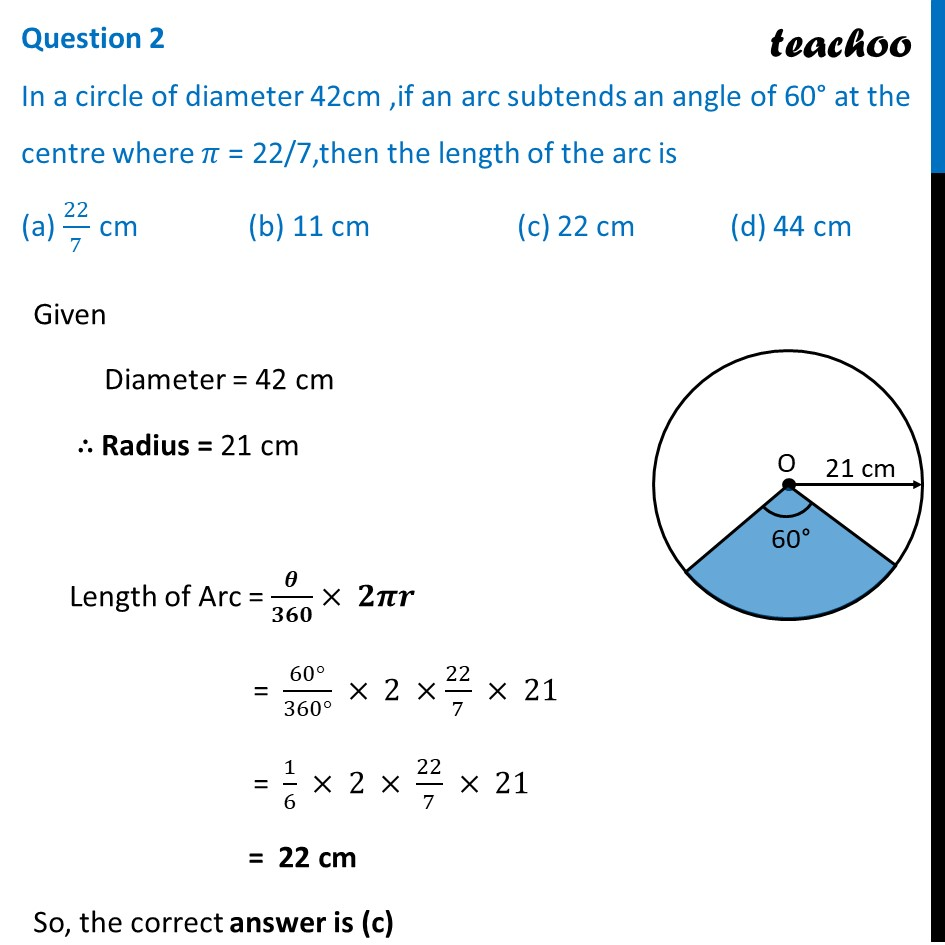CBSE Class 10 Sample Paper for 2022 Boards - Maths Basic [MCQ]

Class 10
Solutions of Sample Papers for Class 10 Boards

## (a) 22/7  cm        (b) 11 cm    (c) 22 cm  (d) 44 cm

This question is inspired from Question 14 - CBSE Class 10 Sample Paper for 2021 Boards - Maths StandardLearn in your speed, with individual attention - Teachoo Maths 1-on-1 Class

### Transcript

Question 2 In a circle of diameter 42cm ,if an arc subtends an angle of 60° at the centre where 𝜋 = 22/7,then the length of the arc is (a) 22/7 cm (b) 11 cm (c) 22 cm (d) 44 cm Given Diameter = 42 cm ∴ Radius = 21 cm Length of Arc = 𝜽/𝟑𝟔𝟎× 𝟐𝝅𝒓 = (60°)/(360°) × 2 ×22/7 × 21 = 1/6 × 2 × 22/7 × 21 = 22 cm So, the correct answer is (c)